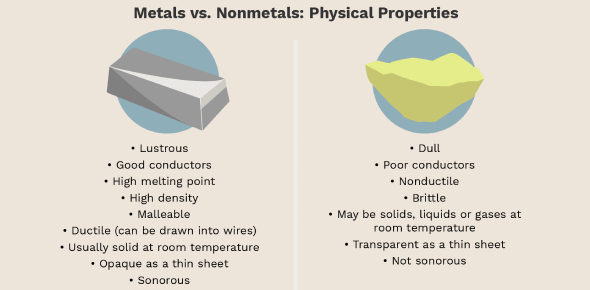# Metals And Non-metals Basic Quiz! Test

15 Questions | Attempts: 6949
ShareSettingsWelcome to the Metals and Non-Metals Basic Quiz. Elements can be described as either metal or nonmetal, with one key difference being how metals look. Most of the nonmetals except for hydrogen can be found on the right side of the periodic table. While metals are good conductors of electricity, nonmetals are not. By taking this quiz, you will get to test out just how well you know the difference between elements that fall between these two groups.

• 1.
An element reacts with oxygen to give a compound with a high melting point. This compound is also soluble in water. The element is likely to be
• A.

Calcium

• B.

Carbon

• C.

Silicon

• D.

Iron

• 2.
Which of the following metal exists in the liquid state?
• A.

Na

• B.

Ag

• C.

Cr

• D.

Hg

• 3.
Which of the following pairs will give displacement reaction?
• A.

NaCl solution and copper metal

• B.

MgCl2 solution and aluminium metal

• C.

FeSO4 solution and silver metal

• D.

AgNO3 solution and copper meta

• 4.
An iron nail is dipped in a copper sulfate solution. It is observed that
• A.

The colour of the solution remains unchanged

• B.

The colour of the solution becomes red

• C.

The colour of the solution turns to light green

• D.

None of these

• 5.
An element A is soft and can be cut with a knife. This is very reactive to air and cannot be kept open in air. It reacts vigorously with water. Identify the element from the following
• A.

Mg

• B.

Na

• C.

P

• D.

Ca

• 6.
An electrolytic cell consists of i. positively charged cathode ii. negatively charged anode iii. positively charged anode iv. negatively charged cathode
• A.

(i) and (ii)

• B.

(iii) and (iv)

• C.

(i) and (iii)

• D.

(ii) and (iv)

• 7.
Which of the following are not ionic compounds? i. KCl ii. HCl iii. CCl4 iv. NaCl
• A.

(i) and (ii)

• B.

(ii) and (iii)

• C.

(iii) and (iv)

• D.

(i) and (iii)

• 8.
What happens when calcium is treated with water? i. It does not react with water ii. It reacts violently with water iii. It reacts less violently with water iv. Bubbles of hydrogen gas formed stick to the surface of calcium
• A.

(i) and (iv)

• B.

(ii) and (iii)

• C.

(i) and (ii)

• D.

(iii) and (iv)

• 9.
Alloys are homogeneous mixtures of a metal with a metal or non-metal. Which among the following alloys contain non-metal as one of its constituents?
• A.

Amalgam

• B.

Brass

• C.

Bronze

• D.

Steel

• 10.
One of the following is not a neutral oxide. This is
• A.

CO

• B.

H2O

• C.
• D.
• 11.
2 mL each of concentrated HC1, HN03, and a mixture of concentrated HC1 and concentrated HN03 in the ratio of 3: 1 was taken in test tubes labeled as A, B, and C. A small piece of metal was put in each test tube. No change occurred in test tubes A and B but the metal got dissolved in test tube C respectively. The metal could be
• A.

Al

• B.

Au

• C.

Cu

• D.

Ag

• 12.
Metal A when dipped in solution of a salt of metal B, then metal B is displaced. this shows that
• A.

Metal A is more reactive than metal B

• B.

Metal B is more reactive than metal A

• C.

Metal A and metal B are equally reactive

• D.

None of these

• 13.
Which of the following metals exist in their native state in nature? i. Cu ii. Au iii. Zn iv. Ag
• A.

(i) and (ii)

• B.

(ii) and (iii)

• C.

(ii) and (iv)

• D.

(iii) and (iv)

• 14.
Metals are refined by using different methods. Which of the following metals are refined by electrolytic refining? i. Au ii. Cu iii. Na iv. K
• A.

(i) and (ii)

• B.

(i) and (iii)

• C.

(ii) and (iii)

• D.

(iii) and (iv)

• 15.
The most abundant Metal in the earth’s crust is
• A.

Al

• B.

K

• C.

Hg

• D.

Ag

## Related TopicsBack to top
×

Wait!
Here's an interesting quiz for you.